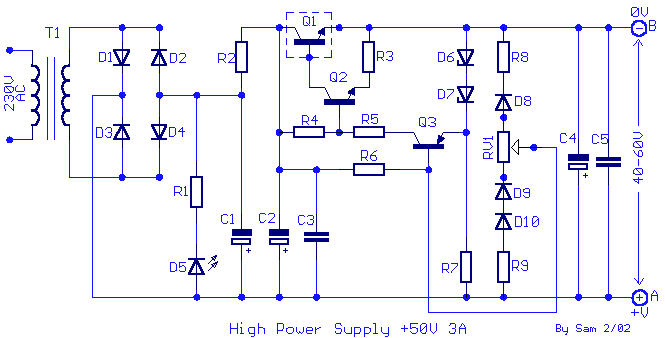# 50V DC 3A Stabilized and Regulated Power SupplyThis is the DC power supply that will give you 50V / 3A output, regulated and stabilized.

Components List:

 R1=10Kohm R8=1.8Kohm 0.5W Q3=BC303 or BC461 R2=1 ohm 5W R9=3.3Kohm 0.5W D1….4=Bridge 15A R3=3.9 ohms 1W RV1=470 ohms pot. D5=LED RED 5mm R4=6.8Kohm 1W C1-2-4=4700uF 100V D6-7=10V 1W Zener R5=390 ohms 1W C3-5=100nF 250V MKT D8-9-10=1N4007 R6=100Kohm 0.5W Q1=2N3055 on heatsink T1=230Vac / 55V 3A R7=1.2Kohm 1W Q2=BD162 or BD243 or BD543

There some times that we need a stabilized , together regulated power supply and relatively high voltage . These specifications cover our circuit. It is a power supply circuit that can give output of +40 V to +60 V 3A, with simultaneous stabilization materials used are very simple and there will be difficulties in manufacturing, it is sufficient to look for certain signs.

1. For smaller output voltages of +50 V to the +40 V, the Q1 is hot enough, so that it needs a large heatsink.
2. For voltages greater than the +50 V to +70 V, the stabilization is not satisfactory.

Conclusion: ideal output voltage +45 V to +60 V. In circuit diagram, the variable resistor / potensiometer of RV1, is used to vary the output voltage between +40 V to +70 V, but maybe we should replace it with two fixed resistors, when finished setting the desired value. The reason is that with the time change occurs output voltage up to 3V with connected potensiometer.

CAUTION ! The positive output corresponds to the point [ A] and the output of 0V, in [ B], which must not be connected to ground .

Power supply is an electronic device that supplies electric energy to an electrical load. The primary function of a power supply is to convert one form of electrical energy to another and, as a result, power supplies are sometimes referred to as electric power converters. Some power supplies are discrete, stand-alone devices, whereas others are built into larger devices along with their loads. Examples of the latter include power supplies found in desktop computers and consumer electronics devices.